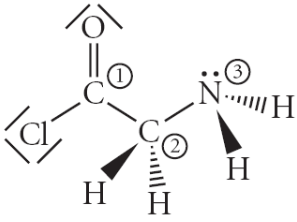# Problem: Consider the molecule below. Determine the molecular geometry at each of the 3 labeled atoms.A) 1 = tetrahedral, 2 = tetrahedral, 3 = tetrahedralB) 1 = trigonal planar, 2 = tetrahedral, 3 = tetrahedralC) 1 = trigonal planar, 2 = trigonal pyramidal, 3 = trigonal pyramidalD) 1 = tetrahedral, 2 = tetrahedral, 3 = trigonal planarE) 1 = trigonal planar, 2 = tetrahedral, 3 = trigonal pyramidal

###### FREE Expert Solution1:

3 atoms around the central atom

AX3 → trigonal planar

2:

4 atoms around the central atom

87% (147 ratings)###### Problem Details

Consider the molecule below. Determine the molecular geometry at each of the 3 labeled atoms.A) 1 = tetrahedral, 2 = tetrahedral, 3 = tetrahedral

B) 1 = trigonal planar, 2 = tetrahedral, 3 = tetrahedral

C) 1 = trigonal planar, 2 = trigonal pyramidal, 3 = trigonal pyramidal

D) 1 = tetrahedral, 2 = tetrahedral, 3 = trigonal planar

E) 1 = trigonal planar, 2 = tetrahedral, 3 = trigonal pyramidal